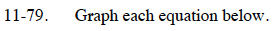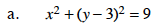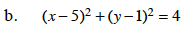Home > CCA2 > Chapter 11 > Lesson 11.2.4 > Problem11-79

11-79.
1. Graph each equation below. Homework Help ✎

1. x2 + (y − 3)2 = 9

2. (x − 5)2 + (y − 1)2 = 4What kind of equation does this look like?

This is the graphing form of a circle equation.
center: (0, 3)